# 图像对比度增强算法

### 一、全局对比度增强1$^1$

#### 1. 直方图均衡化 Histogram Equalization

##### a. 基本思想

- 对于灰度值连续的情况，使用灰度的累积分布函数CDF做转换函数，可以使得输出图像的灰度符合均匀分布。
- 对于灰度值不连续的情况，存在舍入误差，得到的灰度分布大致符合均匀分布。
- 直观地理解，如果某一个灰度范围（如200-201）的像素点很少，那么它的概率密度值就会很小，所以CDF在200-201附近的增长变化就会很小；反之，如果某一个灰度范围（如100-101）的像素点很多，CDF在100-101附近的增长变化会很大。总体来看，以灰度为横轴，CDF为纵轴画曲线。这种向上凸的曲线，很像gamma变换: s=crγ$s=cr^\gamma$γ<1$\gamma<1$的情形。将灰度集中的部分拉伸，而将灰度不集中的部分压缩，达到提高对比度的效果。
- 直方图均衡可以看做自适应的gamma变换或者分段变换。前者的优势在于，不需要指定任何参数，所有运算都是基于图像本身的。

##### b. 算法
1. 根据图像灰度计算灰度概率密度函数PDF$PDF$
2. 计算累积概率分布函数CDF$CDF$
3. CDF$CDF$归一化到原图灰度取值范围，如[0,255]。
4. 之后CDF$CDF$四舍五入取整，得到灰度转换函数sk=T(rk)$s_k=T(r_k)$
5. CDF$CDF$作为转换函数，将灰度为rk$r_k$的点转换为sk$s_k$灰度
##### c. matlab实验

%% 直方图均衡
clear all;clc;close all;
ImgFile='E:\图像处理\冈萨雷斯图片库\DIP3E_Original_Images_CH03\Fig0310(b)(washed_out_pollen_image).tif';
ImgHistEq=histeq(ImgIn,256);
figure;subplot(121);imshow(uint8(ImgIn));title('原图');
subplot(122);imshow(ImgHistEq);title('全局灰度增强 - 直方图均衡');
figure;subplot(121);imhist(ImgIn,256);
axis([0 255 0 1e5]);title('原图的直方图');
subplot(122);imhist(ImgHistEq,256);axis([0 255 0 1e5]);title('直方图均衡化后的直方图');
% 自定义直方图均衡
[counts,x]=imhist(ImgIn,256);
cum_counts=cumsum(counts);
cum_counts=uint8(cum_counts/max(cum_counts)*255);% 转化函数
figure;plot(x,cum_counts);axis([0 255 0 255]);
xlabel('原图灰度');ylabel('转换后灰度');title('原图CDF转化的灰度映射函数');
ImgOut=nan(size(ImgIn));
for i=1:length(x)
ImgOut(ImgIn==x(i))=cum_counts(i);
end
ImgOut=uint8(ImgOut);
figure;imshow(uint8(ImgOut));title('自定义直方图均衡')
figure;imhist(ImgOut,256);axis([0 255 0 1e5]);title('自定义直方图均衡的直方图')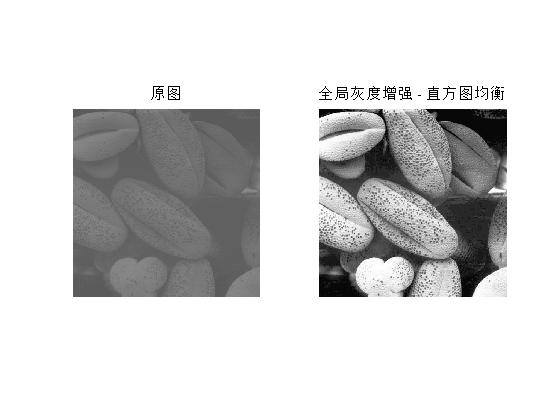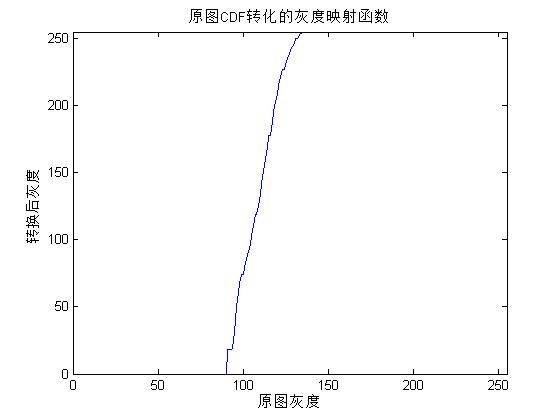#### 2. 直方图匹配 Histogram Matching

##### a. 基本思想

• 对于某些图像，灰度分布过于不均衡，例如背景很大且均匀。直接做直方图均衡会把前景“洗白”，效果很差。
• 直方图匹配需要设定一个直方图分布。不同的图像，此分布可能不同。
###### b. 算法
1. 根据图像计算概率密度分布pr(r)$p_r(r)$
2. 根据pr(r)$p_r(r)$计算累计分布函数sk=T(rk)$s_k=T(r_k)$
3. 根据给定的目标分布pz(z)$p_z(z)$计算累计分布函数G(zq)$G(z_q)$
4. 对于每一个k$k$，找到一个q$q$，使得G(zq)$G(z_q)$约等于sk$s_k$
5. 将原图中灰度为k$k$的点变为灰度q$q$
##### c. Matlab实验
clear all;clc;close all;
ImgFile='E:\图像处理\冈萨雷斯图片库\DIP3E_Original_Images_CH03\Fig0323(a)(mars_moon_phobos).tif';
figure;subplot(121);imshow(ImgIn);title('原图')
subplot(122);imhist(ImgIn);title('原图Hist')
% histeq
ImgHistEq=histeq(ImgIn);
figure;subplot(121);imshow(ImgHistEq);title('Hist EQ');
subplot(122);imhist(ImgHistEq,256);title('Hist EQ')
% 根据3.35(a)估计的分布
x=[0 8 16 180 200 255];
Y=[0 70000 8000 0 3139 0];
xi=0:255;
yi = interp1q(x',Y',xi');
figure;plot(xi,yi,'.');title('目标 Hist')
ImgHistMatch = histeq(ImgIn, yi);
figure;subplot(121);imshow(ImgHistMatch);title('histeq匹配')
subplot(122);imhist(ImgHistMatch,256);title('histeq匹配直方图')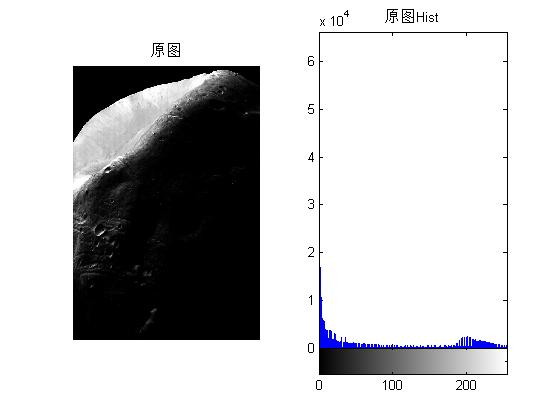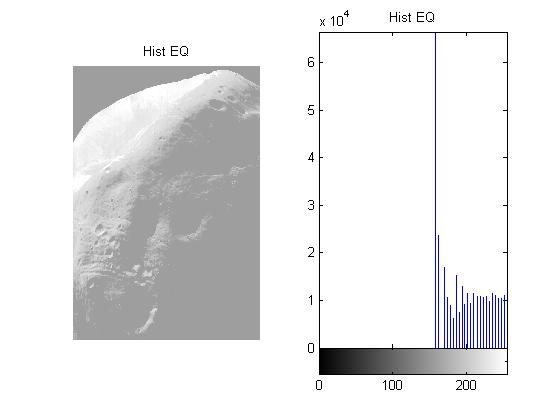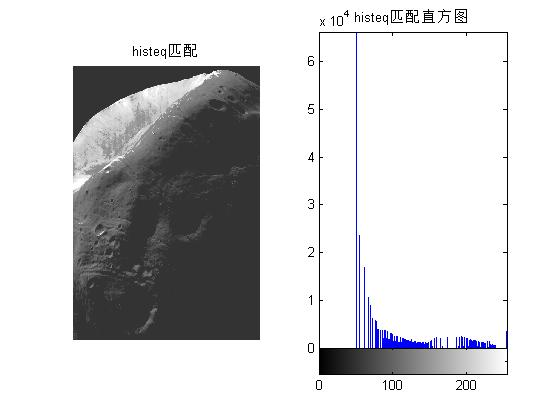%% 自定义匹配
clear all;clc;close all;
ImgFile='E:\图像处理\冈萨雷斯图片库\DIP3E_Original_Images_CH03\Fig0323(a)(mars_moon_phobos).tif';
x=[0 8 16 180 200 255];
Y=[0 70000 8000 0 3139 0];
xi=0:255;
yi = interp1q(x',Y',xi');
[counts, x]=imhist(ImgIn,256);
cum_counts=cumsum(counts);% 累加CDF
% 归一化到[0 255]
cum_counts=cum_counts./numel(ImgIn);
s_k=round(cum_counts*255);
cum_yi=cumsum(yi);% 目标累加CDF
g_z=round(cum_yi./sum(yi)*255);
figure;plot(s_k,'r');hold on;plot(g_z,'g');grid on;hold off;
legend('s_k','g_z')
axis([0 255 0 255]);title('原图累计CDF 和 目标累计CDF')
map=nan(size(s_k));
for k=1:length(s_k)
delta=abs(g_z-s_k(k));
[~,z]=min(delta);
map(k)=z;
end
figure;plot(0:255,map);axis([0 255 0 255]);grid on;
xlabel('输入灰度');ylabel('输出灰度');title('灰度映射曲线')

ImgOut=zeros(size(ImgIn));
for m=0:length(map)-1
ImgOut(ImgIn==m)=map(m+1);
end
ImgOut=uint8(ImgOut);
figure;imshow(ImgOut);title('直方图匹配')
figure;imhist(ImgOut,256);title('直方图匹配');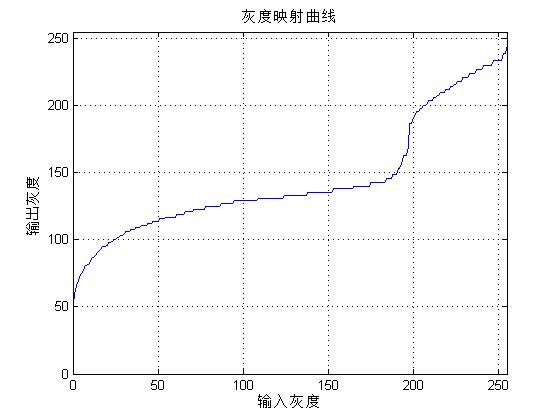### 二、局部对比度增强

#### 3. 邻域统计方法

##### b. 算法
1. 初始化：增强常数E$E$，灰度下阈值k0$k_0$，标准差下阈值 k1$k_1$，标准差上阈值 k2$k_2$，窗口半宽s$s$
2. 计算图像灰度均值MG$M_G$和灰度标准差σG$\sigma_G$
3. 对于每一个像素，计算邻域（大小为2step+1$2*step+1$的方块）内灰度均值ML$M_L$和标准差σL$\sigma_L$
4. 如果ML<=k0MG$M_L<=k_0*M_G$并且k1σG<=σL<=k2σG$k_1*\sigma_G<=\sigma_L<=k_2*\sigma_G$，将像素灰度乘以E$E$
##### c. matlab代码

%% 局部对比度增强
clear all;clc;close all;
ImgFile='E:\图像处理\冈萨雷斯图片库\DIP3E_Original_Images_CH03\tungsten_original.tif';
step=1;
para.E=4.0;
para.k0=0.4; % 均值下阈值
para.k1=0.02; % 标准差下阈值
para.k2=0.4; % 标准差上阈值
ImgHistEq=histeq(ImgIn,256);
ImgIn=double(ImgIn);
% ImgIn=double(rgb2gray(ImgIn));
[ MeanLocal,VarLocal ] = LocalStatistics( ImgIn, step );
[ ImgOut ] = LocalEnhancement( ImgIn, MeanLocal, VarLocal,para );

figure;imshow(uint8(ImgIn));title('原图');
figure;imshow(uint8(ImgOut));title('局部统计增强');
figure;imshow(ImgHistEq);title('全局灰度增强 - 直方图均衡');

function [ ImgOut ] = LocalEnhancement( ImgIn, MeanLocal, VarLocal,para )
%LOCALENHANCEMENT Summary of this function goes here
% 使用局部均值和局部标准差实现局部图像增强
%   Detailed explanation goes here
[rows, cols]=size(ImgIn);
MeanGlobal=mean(ImgIn(:));
VarGlobal=std(ImgIn(:));
for i=1:rows
for j=1:cols
if MeanLocal(i,j)<=para.k0*MeanGlobal...
&&VarLocal(i,j)>=para.k1*VarGlobal...
&&VarLocal(i,j)<=para.k2*VarGlobal
ImgIn(i,j)=para.E*ImgIn(i,j);
end
end
end
ImgOut=ImgIn;
end


function [ MeanLocal,VarLocal ] = LocalStatistics( ImgIn, step )
%LOCALSTATISTICS Summary of this function goes here
% 求局部的灰度均值灰度方差
% ImgIn - 输入灰度图，double
% step  - 窗口大小为2*step+1，step越大，细节越少
%   Detailed explanation goes here
% 矩阵边缘补齐
[rows, cols] = size(ImgIn);
MeanLocal=zeros(size(ImgIn));
VarLocal=zeros(size(ImgIn));
for i = step+1:1:rows-step
for j = step+1:1:cols-step
LocalNeighbor=ImgIn(i-step:i+step,j-step:j+step);
MeanLocal(i,j)=mean(LocalNeighbor(:));
VarLocal(i,j)=std(LocalNeighbor(:));
end
end
% 切割成和原图相同的大小
MeanLocal=MeanLocal(2:end-1,2:end-1);
VarLocal=VarLocal(2:end-1,2:end-1);

end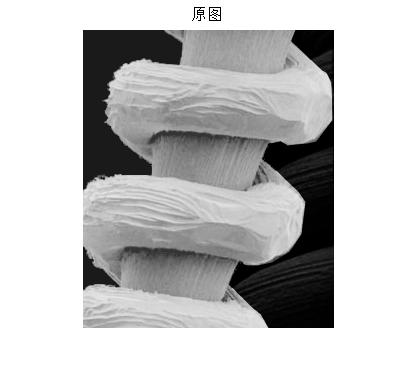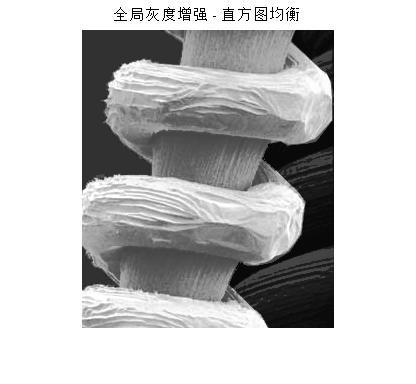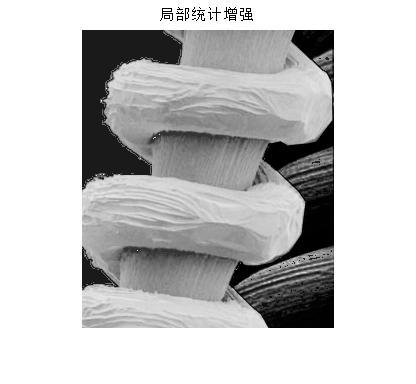2017.4.24补充:

1. 本文主要参考冈萨雷斯《数字图像处理》第三版英文版
2. 如果matlab读入*.tif图片出错，参见：
http://jingyan.baidu.com/article/aa6a2c14f9441b0d4c19c4b0.html